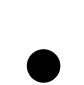Bag Of Cows

## Binary & Hexadecimal Conversion Questions

 Automatic random question generator for various different type of binary and hexadecimal to denary conversion. If you enter any numeric value into the question code box, you will get a particular set of random questions. If you are going to print out the questions, tick 'Written Test' to leave room for showing working. Questions Binary whole numbers * * * Binary addition * * * Binary subtraction * * Binary Multiplication * * Hexadecimal & Denary * * * Hexadecimal & Binary * * * Binary negative numbers (Two's Compliment) * * Binary fractions * * Real numbers (two's compliment mantissa and exponent) *
 Question code: How many questions: Written Test